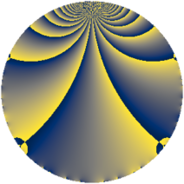# Properties

 Label 2960.1.ezLevel $2960$ Weight $1$ Character orbit 2960.ez Rep. character $\chi_{2960}(1099,\cdot)$ Character field $\Q(\zeta_{12})$ Dimension $8$ Newform subspaces $1$ Sturm bound $456$ Trace bound $0$

# Related objects

## Defining parameters

 Level: $$N$$ $$=$$ $$2960 = 2^{4} \cdot 5 \cdot 37$$ Weight: $$k$$ $$=$$ $$1$$ Character orbit: $$[\chi]$$ $$=$$ 2960.ez (of order $$12$$ and degree $$4$$) Character conductor: $$\operatorname{cond}(\chi)$$ $$=$$ $$2960$$ Character field: $$\Q(\zeta_{12})$$ Newform subspaces: $$1$$ Sturm bound: $$456$$ Trace bound: $$0$$

## Dimensions

The following table gives the dimensions of various subspaces of $$M_{1}(2960, [\chi])$$.

Total New Old
Modular forms 24 24 0
Cusp forms 8 8 0
Eisenstein series 16 16 0

The following table gives the dimensions of subspaces with specified projective image type.

$$D_n$$ $$A_4$$ $$S_4$$ $$A_5$$
Dimension 0 0 8 0

## Trace form

 $$8 q + 8 q^{6} + O(q^{10})$$ $$8 q + 8 q^{6} + 8 q^{10} + 8 q^{11} - 8 q^{14} + 4 q^{16} + 4 q^{19} - 4 q^{21} - 4 q^{34} - 4 q^{35} + 4 q^{39} - 4 q^{44} - 4 q^{46} + 4 q^{49} - 8 q^{51} + 4 q^{56} + 8 q^{60} - 4 q^{61} + 4 q^{65} + 8 q^{66} + 4 q^{69} + 8 q^{71} - 8 q^{74} - 4 q^{76} - 4 q^{81} - 8 q^{84} - 8 q^{85} - 4 q^{91} + 4 q^{96} + O(q^{100})$$

## Decomposition of $$S_{1}^{\mathrm{new}}(2960, [\chi])$$ into newform subspaces

Label Dim. $$A$$ Field Image CM RM Traces $q$-expansion
$$a_2$$ $$a_3$$ $$a_5$$ $$a_7$$
2960.1.ez.a $$8$$ $$1.477$$ $$\Q(\zeta_{24})$$ $$S_{4}$$ None None $$0$$ $$0$$ $$0$$ $$0$$ $$q-\zeta_{24}^{7}q^{2}+\zeta_{24}^{5}q^{3}-\zeta_{24}^{2}q^{4}+\cdots$$